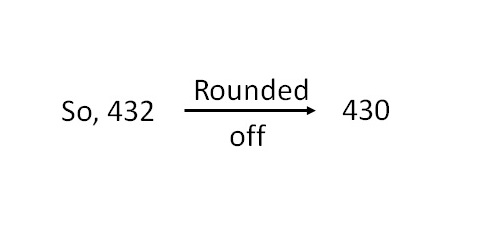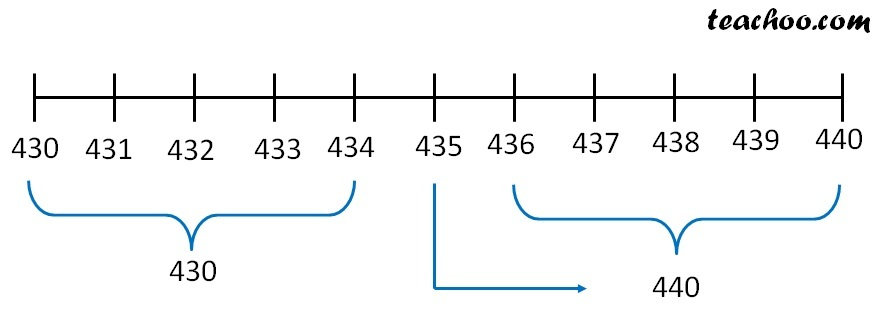Suppose we are given the number

432

Rounding off to nearest tens means

• We write numbers as 430 or 440

Since 430 is near to 432.In general• Numbers 431, 432, 433, 434 will be rounded off to 430.
• Numbers 436, 437, 438, 439 will be rounded off to 440
• Number 435, the middle most number will always be larger, i.e 440

1. Chapter 1 Class 6 Knowing our Numbers
2. Concept wise
3. Estimating and Rounding off

Transcript

Rounding off to nearest ten (10) Suppose we are given the number 432 Rounding off to nearest tens means We write numbers as 430 or 440 Since 430 is near to 432. In general Numbers 431, 432, 433, 434 will be rounded off to 430. Numbers 436, 437, 438, 439 will be rounded off to 440 Number 435, the middle most number will always be larger, i.e 440

Estimating and Rounding off